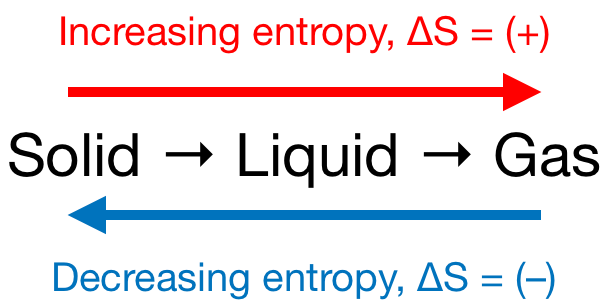# Problem: Predict the sign of the entropy change of the system for each of the following reactions.(a) N2 (g) + 3H2 (g)  →  2NH3 (g)(b) CaCO3(s)  →  CaO(s) + CO2(g)(c) 3C2H2(g)  →  C6H6(g)(d) Al2O3(s) + 3H2 (g)  →  2Al(s) + 3H2O(g)

###### FREE Expert Solution

We’re being asked to predict the sign of the entropy change (ΔS) for the given reactions

Recall that if:

• ΔS > 0 or ΔS = (+); entropy is increased

• ΔS < 0 or ΔS = (–); entropy is decreased

Remember that phase changes lead to a change in entropy:Also, recall that an increase in the number of gas molecules in the reaction also leads to an increase in entropy.

92% (432 ratings)###### Problem Details

Predict the sign of the entropy change of the system for each of the following reactions.
(a) N2 (g) + 3H2 (g)  →  2NH3 (g)
(b) CaCO3(s)  →  CaO(s) + CO2(g)
(c) 3C2H2(g)  →  C6H6(g)
(d) Al2O3(s) + 3H2 (g)  →  2Al(s) + 3H2O(g)